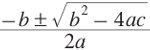## Quadratic Equations - Summary

A polynomial of degree 2 is called quadrilateral polynomial. The general form of a quadrilateral polynomial is ax2 + bx+ c, where a, b, c are real numbers such that a ≠ 0 and x is a real variable.

If p(x) = ax2  + bx + c, a ≠ 0 is a quadratic polynomial and α is a real number, then p(α) = aα2 + bα + c is known as the value of the quadratic polynomial p(x).

A real number α is said to be a zero of the quadratic polynomial p(x) = ax2  + bx + c, if p(α) = 0.

If p(x) = ax2  + bx + c is a quadratic polynomial,then p(x) = 0 i.e. ax2  + bx + c = 0, a ≠ 0 is called a quadratic equation.

A real number α is said to be root of the quadratic equation ax2  + bx + c = 0, if aα2 + bα + c = 0.
In other words, α is a root of ax2  + bx + c = 0 if and only if α is zero pf the polynomial p(x) = ax2  + bx + c.

If ax2  + bc + c, a ≠ 0 is factorizable into product of two linear factors, then roots of the quadratic equation can be found by equating each factor to zero.

The roots of a quadratic equation can be found by using the method of completing the square.

The roots of the quadratic equation ax2  + bc + c, a ≠ 0 can be found by using the quadratic formulaprovided, b2 - 4ac ≥ 0.

Nature of the roots of quadratic equation ax2 + bx + c = 0, a ≠ 0 depends upon the value of D = b2 - 4ac, which is known as discriminate of the quadratic equation.

The quadratic equation ax2 + bx + c = 0, a ≠ 0 has:
⒜ Two distinct real roots, if D = b2 - 4ac > 0
⒝ Two equal roots i.e. coincident real roots if D = b2 - 4ac = 0
⒞ No real roots, if D = b2 - 4ac < 0.

Greek mathematician Euclid developed a geometrical approach for finding out lengths
which in our present day terminology, are solutions of quadratic equations.

Solving quadratic equations in general form is often credited to ancient Indian Mathematicians. In fact, Brahma Gupta (A.D 598 - 665) gave an explicit formula to solve a quadratic equation of the form ax2 + bx = c . Later Sridhar Acharya (1025 A.D) derived a formula, now known as the quadratic formula, (as quoted by Bhaskara II) for solving a quadratic equation by the method of completing the square.#### Post a Comment

We love to hear your thoughts about this post!